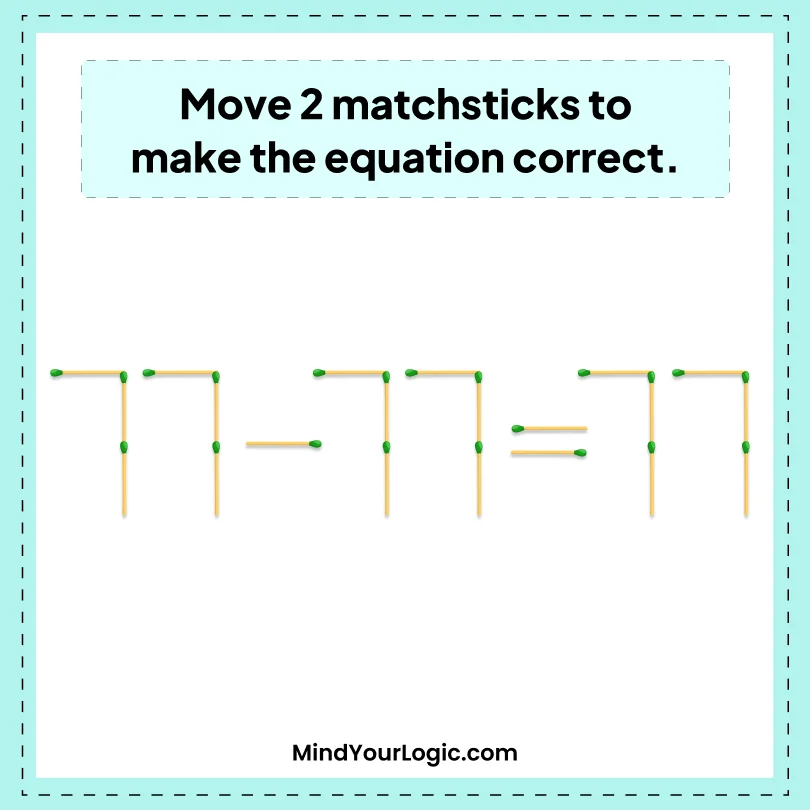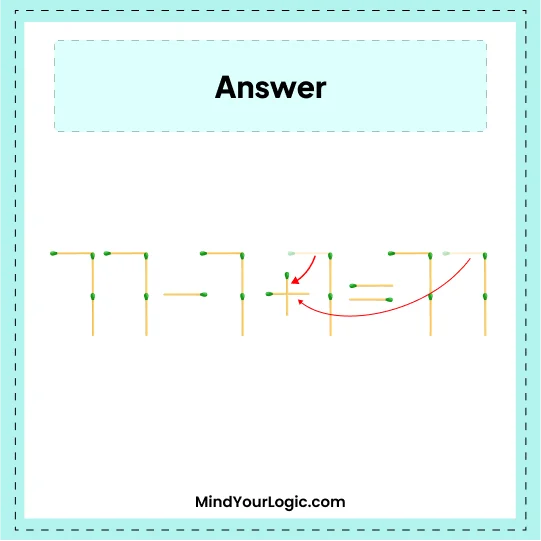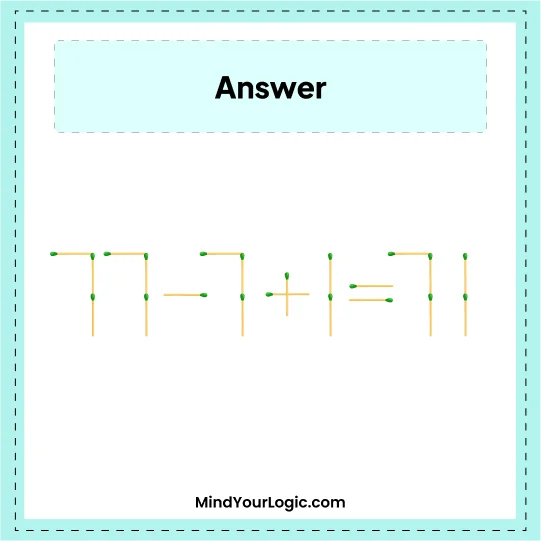# 77 - 77 = 77 - Matchstick Puzzles

###### 8.Matchstick Puzzles
`Move 2 matchsticks to make the equation correct.`•

Explanation :

` Take one stick from right-hand side 77 and one stick from middle 77 and create a + sign in between the middle 77, which becomes 7+1   the correct equation we get is 77 - 7 + 1 = 71.`